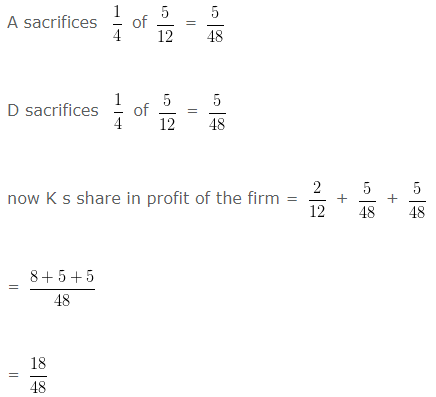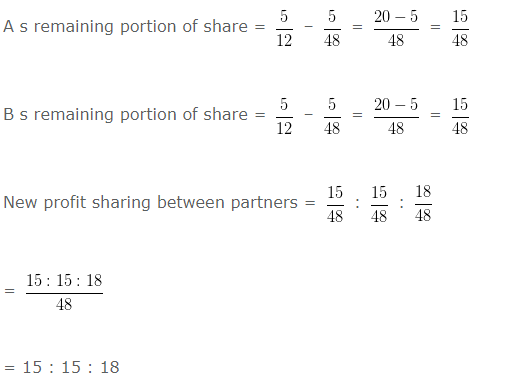# Sacrificing Ratio in Partnership Accounting

Sacrificing ratio in partnership accounting – The ratio in which an existing partner is ready to sacrifice his share of profit to another partner is known as sacrificing ratio.

The formula for sacrificing ratio is :

Sacrificing Ratio = New Ratio – Old ratio

### Sacrificing Ratio in Partnership Accounting : Example 1

A , D and K are partners sharing profits and losses in the ratio of 5 : 5 : 2 respectively. A sacrifices 1/4 of his share  and D sacrifices 1/4 of his share in favour of K .What will be the sacrificing ratio between partners.

Explanation : –Sacrificing ratio = Old ratio – New ratio

A = 5/12 – 15/48

= (20-15)/48

= 5/48

D = 5/12 – 15/48

= (20-15)/48

= 5/48

5 : 5

### Sacrificing Ratio in Partnership Accounting : Example 2

Abra and Dabra are partners sharing profits and losses in the ratio of 6 5 respectively. A new partner Cabra
is admitted into partnership for 10 % share in prots.What will be the sacrificing ratio between partners?

Explanation : –

Let total prot of the firm = 1

is admitted into partnership for s share = 10% = 10/100 = 1/10

Remaining prot after admission of is admitted into partnership for = 1 – (1/10)

= (10-1)/10

= 9/10

Abra will get 6/11 of 9/10 = 54/110

Dabra will get 5/11 of 9/10 = 45/110

New profit sharing ratio between Abra , Dabra and is admitted into partnership for

= 54/110 : 45/110 : 1/10

= (54:45:11)/110

= 54 : 45 : 11

Sacrificing ratio = Old ratio – New ratio

Abra = (6/11) – (54/110)

= (60-54)/110

= 6/110

Dabra = (5/11) – (45/110)

= (50-45)/110

= 5/110

Sacrificing ratio = 6 : 5

### Sacrificing Ratio in Partnership Accounting : Example 3

Good and Better are partners sharing profits and losses in the ratio of 7 and 5 respectively. A new partner
Best is admitted into partnership for 10 % share in prots.What will be the sacrificing ratio between partners?

Explanation : –

Let total profit of the firm = 1

Best s share = 10% = 10/100 = 1/10

Remaining prot after admission of Best = 1 – (1/10)

= (10-1)/10

= 9/10

Good will get 7/12 of 9/10 = 63/120

Better will get 5/12 of 9/10 = 45/120

New profit sharing ratio between Good , Better and Best

= 63/120 : 45/120 : 1/10

= (63:45:12)/120

= 63:45:12

Sacrificing ratio = Old ratio – New ratio

Good = (7/12) – (63/120)

= (70-63)/120

= 7/120

Better = (5/12) – (45/120)

= (50-45)/20

= 5/120

Sacrificing ratio = 7 : 5

### Sacrificing Ratio in Partnership Accounting : Example 4

Kaya and Maya are partners sharing profits and losses in the ratio of 5 and 3 respectively. A new partner
Saya is admitted into partnership for 40 % share in prots.What will be the sacrificing ratio between partners?

Explanation : –

Let total prot of the firm = 1

Saya s share = 40% = 40/100 = 4/10

Remaining prot after admission of Saya = 1 – (4/10)

= (10-4)/10

= 6/10

Kaya will get 5/8 of 6/10 = 30/80

Maya will get 3/8 of 6/10 = 18/80

New profit sharing ratio between Kaya , Maya and Saya

= (30/80) : (18/80) : (4/10)

= (30:18:32)/80

= 30:18:32

Sacrificing ratio = Old ratio – New ratio

Kaya = 5/8 – 30/80

= (50-30)/80

= 20/80

Maya = (3/8) – (18/80)

= (30-18)/80

= 12/80

Sacrificing ratio = 20 : 12